Courses

# Systems of Linear Equations, Matrix Algebra & Transform Theory - MCQ Test - 1

## 20 Questions MCQ Test Topicwise Question Bank for Electronics Engineering | Systems of Linear Equations, Matrix Algebra & Transform Theory - MCQ Test - 1

Description
This mock test of Systems of Linear Equations, Matrix Algebra & Transform Theory - MCQ Test - 1 for GATE helps you for every GATE entrance exam. This contains 20 Multiple Choice Questions for GATE Systems of Linear Equations, Matrix Algebra & Transform Theory - MCQ Test - 1 (mcq) to study with solutions a complete question bank. The solved questions answers in this Systems of Linear Equations, Matrix Algebra & Transform Theory - MCQ Test - 1 quiz give you a good mix of easy questions and tough questions. GATE students definitely take this Systems of Linear Equations, Matrix Algebra & Transform Theory - MCQ Test - 1 exercise for a better result in the exam. You can find other Systems of Linear Equations, Matrix Algebra & Transform Theory - MCQ Test - 1 extra questions, long questions & short questions for GATE on EduRev as well by searching above.
QUESTION: 1

### The system of linear equations 4x + 2y = 7 2x + y = 6              has

Solution:

(b) This can be written as AX = B Where A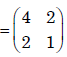Angemented matrix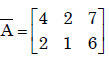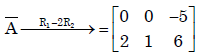rank(A) ≠ rank(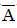). The system is inconsistant .So system has no solution.

QUESTION: 2

### For the following set of simultaneous equations: 1.5x – 0.5y = 2 4x + 2y + 3z = 9 7x + y + 5z = 10

Solution:

(a)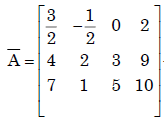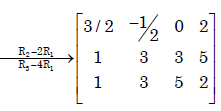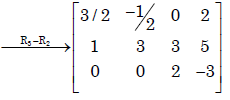∴ rank of() = rank of(A) = 3

∴ The system has unique solution.

QUESTION: 3

### The following set of equations has 3 x + 2 y + z = 4 x – y + z = 2 ​-2 x + 2 z = 5

Solution:

(b)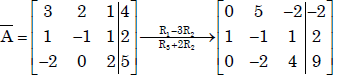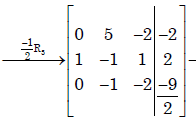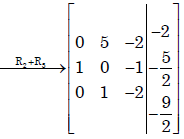∴ rank (A) = rank () = 3
∴ The system has unique solution

QUESTION: 4

Consider the system of simultaneous equations
x + 2y + z = 6
2x + y + 2z =  6
x + y +  z = 5
This system has

Solution:

(c )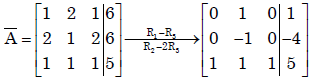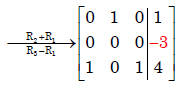∴ rank(A) = 2 ≠ 3 = rank() .

∴ The system is inconsistent and has no solution.

QUESTION: 5

Multiplication of matrices E and F is G. Matrices E and G are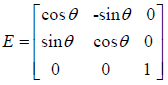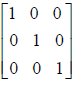What is the matrix F?

Solution:

(c)
Given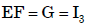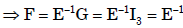QUESTION: 6

Consider a non-homogeneous system of linear equations representing mathematically an over-determined system. Such a system will be

Solution:

Ans.(b)
In an over determined system having more equations than variables, it is necessary to have consistent having many solutions .

QUESTION: 7

For the set of equations
x1 + 2x  + x3 + 4x4 = 0
3x1 + 6x2 + 3x3 + 12x4 = 0

Solution:

given set of equations are x1+2x2+x3+4x4=2 , 3x1+6x2+3x3+12x4=6

consider AB =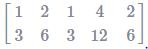⇒ R2 → R2 - 3R1

AB =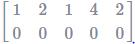P(A)=1; P(AB)=1;n=4

⇒ P(A) =P(B) < no. of variables

⇒ Infinitely many solutions ⇒multiple non-trivial solution

QUESTION: 8

Let P ≠ 0 be a 3 × 3 real matrix. There exist linearly independent vectors x and y such that Px = 0 and Py = 0. The dimension of the range space of P is

Solution:

(b)

QUESTION: 9

The eigen values of a skew-symmetric matrix are

Solution:

(c)

QUESTION: 10

The rank of a 3×3 matrix C (=AB), found by multiplying a non-zero column matrix Aof size 3×1 and a non-zero row matrix B of size 1×3, is

Solution:

(b)

Let A =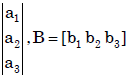Then C = AB =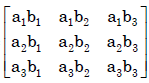Then det (AB) = 0.

Then also every minor
of order 2 is also zero.
∴ rank(C) =1.

QUESTION: 11

Match the items in columns I and II.
​Column I                                               Column II
P. Singular matrix                               1. Determinant is not defined
Q. Non-square matrix                          2. Determinant is always one
R. Real symmetric                              3. Determinant is zero
S. Orthogonal matrix                           4. Eigenvalues are always real
5. Eigenvalues are not defined

Solution:

(a) (P) Singular matrix → Determinant is zero
(Q) Non-square matrix → Determinant is not defined
(R) Real symmetric → Eigen values are always real
(S) Orthogonal → Determinant is always one

QUESTION: 12

Real matrices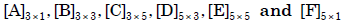are given. Matrices [B] and
[E] are symmetric.
Following statements are made with respect to these matrices.
1. Matrix product [F]T [C]T [B] [C] [F] is a scalar.
2. Matrix product [D]T [F] [D] is always symmetric.
With reference to above statements, which of the following applies?

Solution:

(a)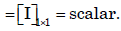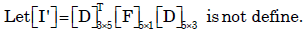QUESTION: 13

The product of matrices (PQ)–1 P is

Solution:

(b)
(PQ) -1 = P Q-1P-1P = Q-1

QUESTION: 14

The matrix A
=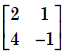is decomposed into a product of a lower triangular matrix [L] and an upper triangular matrix [U]. The properly decomposed [L] and [U] matrices respectively are

Solution:

Ans. (d)

QUESTION: 15

The inverse of the matrix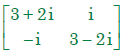is

Solution:

(b)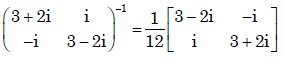QUESTION: 16

The inverse of the 2 × 2 matrix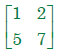is,

Solution:

(a).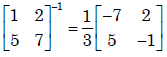QUESTION: 17

There are two containers, with one containing 4 Red and 3 Green balls and the other containing 3 Blue and 4 Green balls. One ball is drawn at random form each container.The probability that one of the ball is Red and the other is Blue will be

Solution: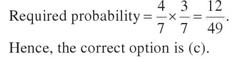QUESTION: 18

The Fourier transform of x(t) = e–at u(–t), where u(t) is the unit step function,

Solution:

Ans. (d)

QUESTION: 19

Given that F(s) is the one-sided Laplace transform of f(t), the Laplace transform of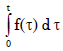is [EC:

Solution:

Ans. (b)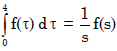……(Lapalace formule)

QUESTION: 20

If f(t) is a finite and continuous function for t, the Laplace transformation is given by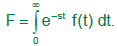For f(t) = cos h mt, the Laplace transformation is…..

Solution: